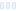# Formula 50 Grape

## vitaminwater - Glaceau

Main info:

Formula 50 Grape
vitaminwater - Glaceau
8 fl oz
50.0 Calories
13.0 g
0 g
0 g

0 g
0 mg
0 g
0 mg
0 g
0 g

Percent calories from...
Nutrition Facts
For a Serving Size of (g)
How many calories are in Formula 50 Grape? Amount of calories in Formula 50 Grape: Calories Calories from Fat (%)
% Daily Value *
How much fat is in Formula 50 Grape? Amount of fat in Formula 50 Grape: Total Fat
How much sodium is in Formula 50 Grape? Amount of sodium in Formula 50 Grape: Sodium
How many carbs are in Formula 50 Grape? Amount of carbs in Formula 50 Grape: Carbohydrates
How many net carbs are in Formula 50 Grape? Amount of net carbs in Formula 50 Grape: Net carbs
How much fiber is in Formula 50 Grape? Amount of fiber in Formula 50 Grape: Fiber
How much glucose is in Formula 50 Grape? Amount of glucose in Formula 50 Grape: Glucose
How much protein is in Formula 50 Grape? Amount of protein in Formula 50 Grape: Protein
Vitamins and minerals
How much Vitamin A is in Formula 50 Grape? Amount of Vitamin A in Formula 50 Grape: Vitamin A
How much Vitamin C is in Formula 50 Grape? Amount of Vitamin C in Formula 50 Grape: Vitamin C
How much Calcium is in Formula 50 Grape? Amount of Calcium in Formula 50 Grape: Calcium
How much Iron is in Formula 50 Grape? Amount of Iron in Formula 50 Grape: Iron
Fatty acids
Amino acids
* The Percent Daily Values are based on a 2,000 calorie diet, so your values may change depending on your calorie needs.Loading similar foods...
Note: Any items purchased after clicking our Amazon buttons will give us a little referral bonus. If you do click them, thank you!Be cool

- Zen orangeI never skip arm day

- Buff broccoli